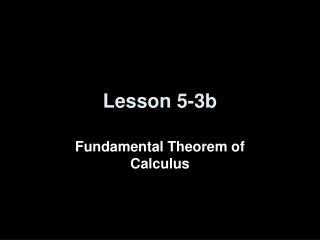DownloadDownload PresentationLesson 5-3b

# Lesson 5-3b

Download Presentation## Lesson 5-3b

- - - - - - - - - - - - - - - - - - - - - - - - - - - E N D - - - - - - - - - - - - - - - - - - - - - - - - - - -
##### Presentation Transcript

1. Lesson 5-3b Fundamental Theorem of Calculus

2. Quiz █ ∫ • Homework Problem: (3ex+ 7sec2x) dx • Reading questions: Fill in the squares below ∫ = 3ex + 7tan x + c █ f(x) dx = F(█) – F(█) If F’(x) = f(x) b b a a

3. Objectives • Understand both forms of the Fundamental Theorem of Calculus • Understand the relation between integration and differentiation

4. Vocabulary • Definite Integral – is a number, not a function

5. lim ∆y ∆x = area = f(x) dx ∆y lim ---- = mt ∆x ∆x→0 ∆x→0 Differentiation vs Definite Integration ∆x ∆x ∆x ∆x ∆y ∆y ∆y ∆y Tangent Line Secant Line Area under the curve Area of rectangle a b a b a b a b area = ∆y ∆x = f(b) (b-a) ∆y f(b) – f(a) slope = ------ = -------------- ∆x b – a ∆y slope ≈ ------- ∆x area ≈ ∆y ∆x b ∫ = f’(a) a

6. Fundamental Theorem of Calculus, Part 1 If f is continuous on [a,b], then the function g defined by is continuous on [a,b], differentiable on (a,b) and g’(x) = f(x) Your book has a proof of this if you are interested. We can see g’(x) = f(x) by using derivatives and FTC part 2. x ∫ a g(x) = f(t) dt a ≤ x ≤ b

7. Fundamental Theorem of Calculus Combining parts 1 and 2 of the FTC, we get F(x) is a function of x and F(a) is just some constant value. Now when we do the following: x x ∫ ∫ a a g(x) = f(t) dt = F(x) – F(a) d ---- dx g(x) = f(t) dt = F(x) – F(a) = g’(x) = = F’(x) – 0 F’(x) = f(x) g’(x) = f(x)

8. Example Problems x ∫ 1) t² dt Find the derivative of each of the following: x 1 ∫ • t3/2 • ------------ dt t² + 17 2 We can’t do what we did in #1! Use the pattern: t=x ⅓t³ | [⅓x³ ] – [⅓1³ ] d(⅓x³ – ⅓) / dx = x² See any pattern? t=1 x3/2 ------------ x² + 17

9. Example Problems with TI-89 x ∫ 1) t² dt Find the derivative of each of the following: Can we use our calculator here? YES!! Hit F3 select derivatives; hit F3 select integration; type in function (t²), integrate with respect to (t), lower limit of integration (1), upper limit of integration (x); close ). Type , and differentiate with respect to x and close ). Should look like this: d( F3 ∫(t^2,t,1,x),x) 1 = x²

10. Example Problems cont 4 ∫ 3) tan²(t) cot (t) dt Find the derivative of each of the following: π/4 x ∫ t tan (t)dt x We can’t do what we did in #1! Use the pattern: We can’t do what we did in #1! Use the pattern: - tan²(x) cot(x) Negative because oflocation of x in integral -x tan (x) Negative because oflocation of x in integral

11. ∫ 6) √2 + sin (t) dt 2 √2 + sin (x²) (2x) Remember Chain Rule Example Problems cont 2x ∫ 5) 5t sin (t) dt Find the derivative of each of the following: 1 We can’t do what we did in #1! Use the pattern: We can’t do what we did in #1! Use the pattern: 10x sin(2x) (2) Remember Chain Rule

12. √1 + (x³)4 (3x2) - √1 + x4 Remember F(b) – F(a) and chain Rule Example Problems cont x³ ∫ 7) √1 + t4dt Find the derivative of each of the following: cos x x ∫ 8) t² dt sin x We can’t do what we did in #1! Use the pattern: We can’t do what we did in #1! Use the pattern: cos²x (-sinx) - sin²x(cosx) Remember F(b) – F(a)and Chain Rule

13. Summary & Homework • Summary: • Definite Integrals are a number • Evaluated at endpoints of integration • Derivative of the integral returns what we started with (with Chain Rule) • Homework: • Day One: pg 402-404: 19, 22, 27, 28, • Day Two: pg 402-404: 3, 7, 9, 61 (see appendix E)

14. Second Set Example Problems x² ∫ 1) sin³(t) dt Find the derivative of each of the following: 1 We can’t do what we did in #1! Use the pattern: sin³(x²) (2x) Remember Chain Rule

15. Second Set Example Problems 5 ∫ 2) (1/t²)dt x We can’t do what we did in #1! Use the pattern: - (1/x²) Negative because oflocation of x in integral Find the derivative of each of the following:

16. Second Set Example Problems √1 + (x²)4 (2x) - √1 + x4 Remember F(b) – F(a) and chain Rule x² ∫ 3) √1 + t4 dt x We can’t do what we did in #1! Use the pattern: Find the derivative of each of the following:

17. Last Example Problem x ∫ t – 3 ----------- dt for -∞ < x < ∞ t² + 7 0 Consider F (x) = Find F (x). For what number x does F attain its minimum value? ______ Justify your answer. How many inflection points does the graph of F have? _____ Justify your answer. x – 3 F’(x) = ----------- x² + 7 When F’(x) = 0 so x = 3First Derivative Test: - 0 + therefore a min When F’’(x) = 0 so x = -1, x = 7